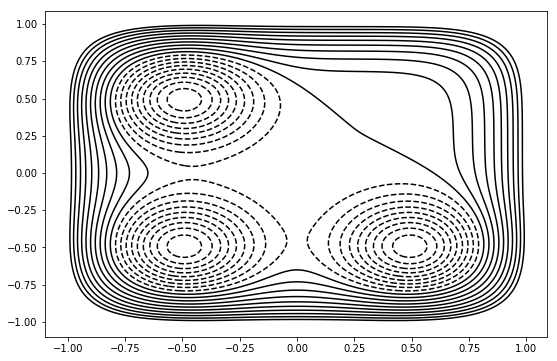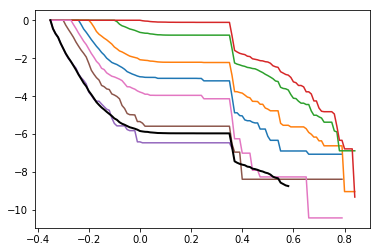# Multiple Interface Set TIS on a Toy Model

This example demostrates multiple interface set TIS on a two dimensional toy model. It consists of thre notebooks: one to setup and run the sampling, one to calculate the flux, and one to analyze the results. In MISTIS, the flux must be performed as a separate calculation.

toy_mistis_1_setup_run
In :
from __future__ import print_function
%matplotlib inline
import openpathsampling as paths
import numpy as np

# we use the %run magic because this isn't in a package
%run ../resources/toy_plot_helpers.py

In :
import openpathsampling.engines.toy as toys

pes = (
toys.OuterWalls([1.0, 1.0], [0.0, 0.0]) +
toys.Gaussian(-1.0, [12.0, 12.0], [-0.5, 0.5]) +
toys.Gaussian(-1.0, [12.0, 12.0], [-0.5, -0.5]) +
toys.Gaussian(-1.0, [12.0, 12.0], [0.5, -0.5])
)

topology=toys.Topology(
n_spatial = 2,
masses =[1.0, 1.0],
pes = pes
)

integ = toys.LangevinBAOABIntegrator(dt=0.02, temperature=0.1, gamma=2.5)

options={
'integ' : integ,
'n_frames_max' : 5000,
'n_steps_per_frame' : 1
}

toy_eng = toys.Engine(
options=options,
topology=topology
)
toy_eng.initialized = True

template = toys.Snapshot(
coordinates=np.array([[-0.5, -0.5]]),
velocities=np.array([[0.0,0.0]]),
engine=toy_eng
)

toy_eng.current_snapshot = template
paths.PathMover.engine = toy_eng

In :
plot = ToyPlot()
plot.contour_range = np.arange(-1.5, 1.0, 0.1)
plot.plot()

Out:In [ ]:
def xval(snapshot):
return snapshot.xyz

def xprime(snapshot):
# this only exists until we set up the ability for the order parameter to decrease
return -snapshot.xyz

def yval(snapshot):
return snapshot.xyz

cvX = paths.FunctionCV(name="cvX", f=xval).with_diskcache()
cvY = paths.FunctionCV(name="cvY", f=yval).with_diskcache()
cvXprime = paths.FunctionCV(name="cvXprime", f=xprime).with_diskcache()

In [ ]:
x_under_min = paths.CVDefinedVolume(cvX, float("-inf"), -0.35)
x_over_max = paths.CVDefinedVolume(cvX, 0.35, float("inf"))
y_under_min = paths.CVDefinedVolume(cvY, float("-inf"), -0.35)
y_over_max = paths.CVDefinedVolume(cvY, 0.35, float("inf"))

stateA = (x_under_min & y_under_min).named("A")
stateB = (x_over_max & y_under_min).named("B")
stateC = (x_under_min & y_over_max).named("C")

In [ ]:
#plot.add_states([stateA, stateB, stateC])
#plot.plot()

In [ ]:
interfacesAB = paths.VolumeInterfaceSet(cvX, float("-inf"), [-0.35, -0.3, -0.27, -0.24, -0.2, -0.1])
interfacesAC = paths.VolumeInterfaceSet(cvY, float("-inf"), [-0.35, -0.3, -0.27, -0.24, -0.2, -0.1, 0.0])
interfacesBA = paths.VolumeInterfaceSet(cvXprime, float("-inf"), [-0.35, -0.3, -0.27, -0.24, -0.2, -0.1])

In [ ]:
ms_outer = paths.MSOuterTISInterface.from_lambdas(
{iface: 0.0 for iface in [interfacesAB, interfacesBA]}
)
network = paths.MISTISNetwork(
[(stateA, interfacesAB, stateB),
(stateA, interfacesAC, stateC),
(stateB, interfacesBA, stateA)],
ms_outers=ms_outer,
strict_sampling=True
)

In [ ]:
scheme = paths.DefaultScheme(network, engine=toy_eng)

In [ ]:
tisAB = network.transitions[(stateA, stateB)]
tisAC = network.transitions[(stateA, stateC)]
tisBA = network.transitions[(stateB, stateA)]

In [ ]:
import logging.config
logging.config.fileConfig("../resources/debug_logging.conf", disable_existing_loggers=False)

In [ ]:
snapA = toys.Snapshot(
coordinates=np.array([[-0.5, -0.5]]),
velocities=np.array([[0.5, 0.0]])
)
init_AB = paths.FullBootstrapping(
transition=tisAB,
snapshot=snapA,
engine=toy_eng,
forbidden_states=[stateC],
extra_ensembles=network.ms_outers
).run()

In [ ]:
snapA = toys.Snapshot(
coordinates=np.array([[-0.5, -0.5]]),
velocities=np.array([[0.0, 0.5]])
)
init_AC = paths.FullBootstrapping(
transition=tisAC,
snapshot=snapA,
engine=toy_eng,
forbidden_states=[stateB]
).run()

In [ ]:
snapB = toys.Snapshot(
coordinates=np.array([[0.5, -0.5]]),
velocities=np.array([[-0.5, 0.0]])
)
init_BA = paths.FullBootstrapping(
transition=tisBA,
snapshot=snapB,
engine=toy_eng,
forbidden_states=[stateC]
).run()

In [ ]:
initial_trajectories = [s.trajectory for s in list(init_AB)+list(init_AC)+list(init_BA)]

In [ ]:
plot.plot(initial_trajectories)

In [ ]:
sset = scheme.initial_conditions_from_trajectories(initial_trajectories)
print(scheme.initial_conditions_report(sset))

In [ ]:
plot.plot([s.trajectory for s in sset])

In [ ]:
minus_samples = []
for minus in network.minus_ensembles:
samp = minus.extend_sample_from_trajectories(
trajectories=sset,
replica=-network.minus_ensembles.index(minus)-1,
engine=toy_eng
)
minus_samples.append(samp)
print(minus_samples)
print(network.minus_ensembles)
sset = sset.apply_samples(minus_samples)
print(scheme.initial_conditions_report(sset))

In [ ]:
# The next two cells are for tests.
# This cell creates initial conditions that will pass analysis on low data.
# The next cell undoes that to use a better initial condition in practice.
better_initial_conditions = sset

for transition in network.sampling_transitions:
outermost_traj = sset[transition.ensembles[-1]].trajectory
for ensemble in transition.ensembles:
original = sset[ensemble]
sample = paths.Sample(replica=original.replica,
ensemble=ensemble,
trajectory=outermost_traj)
sset = sset.apply_samples(sample)

In [ ]:
# NBVAL_SKIP
# tests should not run this, users should. Undoes the previous cell
sset = better_initial_conditions

In [ ]:
plot.plot([s.trajectory for s in sset])

In [ ]:
#logging.config.fileConfig("../resources/debug_logging.conf", disable_existing_loggers=False)
storage = paths.Storage("mistis.nc", "w")
storage.save(template)

In [ ]:
mistis_calc = paths.PathSampling(
storage=storage,
move_scheme=scheme,
sample_set=sset
)
mistis_calc.save_frequency = 100

In [ ]:
#import logging.config
#logging.config.fileConfig("../resources/logging.conf", disable_existing_loggers=False)
mistis_calc.run(100)

In [ ]:
# NBVAL_SKIP
# skip this during testing; leave for full calculation
mistis_calc.run_until(100000)

In [ ]:



toy_mistis_2_flux
In [ ]:
%matplotlib inline
import openpathsampling as paths
import numpy as np


As always, we load things from files so we don't have to set them up again.

In [ ]:
old = paths.Storage("mistis.nc", 'r')
engine = old.engines
network = old.networks
states = set(network.initial_states + network.final_states)

In [ ]:
# must ensure that the diskcache is disabled in order to save,
# otherwise it looks for things that aren't there!
cvs = old.cvs[:]
for cv in cvs:
cv.disable_diskcache()


The flux_pairs variable is a list of 2-tuples, where the first element is the state we're calculating the flux out of, and the second element is the interface we're calculating the flux through.

In [ ]:
flux_pairs = [(t.stateA, t.interfaces) for t in network.transitions.values()]


Set up the simulation and run it!

In [ ]:
sim = paths.DirectSimulation(
storage=None,
engine=engine,
states=states,
flux_pairs=flux_pairs,
initial_snapshot=old.snapshots
)

In [ ]:
%%time
sim.run(150000) # 30 sec
#sim.run(1500000) # 6 min
#sim.run(15000000) # 60 min
#sim.run(150000000) # 10 hr
#sim.run(800000000) # 2 days


Now we move on to the analysis.

In [ ]:
sim.rate_matrix

In [ ]:
sim.n_transitions

In [ ]:
fluxes = sim.fluxes
for f in fluxes:
print f.name, f.name, fluxes[f]

In [ ]:
sim.n_flux_events

In [ ]:
sim.results

In [ ]:
output = paths.Storage("direct_simulation.nc", 'w')
output.save(old.snapshots)
output.save(sim)
output.tag['direct_results'] = sim.results
output.close()


toy_mistis_3_analysis
In :
from __future__ import print_function
# if our large test file is available, use it. Otherwise, use file generated from toy_mistis_1_setup_run.ipynb
import os
test_file = "../toy_mistis_1k_OPS1.nc"
filename = test_file if os.path.isfile(test_file) else "mistis.nc"

In :
print(filename)

../toy_mistis_1k_OPS1.nc

In :
%matplotlib inline
import matplotlib.pyplot as plt
import numpy as np
import openpathsampling as paths

In :
%%time
storage = paths.AnalysisStorage(filename)

CPU times: user 617 ms, sys: 108 ms, total: 725 ms
Wall time: 788 ms

In :
mistis = storage.networks.load(0)

---------------------------------------------------------------------------
NameError                                 Traceback (most recent call last)
<ipython-input-5-6044c40634e8> in <module>

NameError: name 'storage' is not defined
In :
mistis.hist_args['max_lambda'] = { 'bin_width' : 0.01, 'bin_range' : (-0.35, 0.5) }
mistis.hist_args['pathlength'] = { 'bin_width' : 5, 'bin_range' : (0, 150) }

In :
%%time
scheme = storage.schemes
scheme.move_summary(storage.steps)

pathreversal ran 25.354% (expected 25.38%) of the cycles with acceptance 22007/25354 (86.80%)
ms_outer_shooting ran 2.505% (expected 2.54%) of the cycles with acceptance 1484/2505 (59.24%)
shooting ran 48.313% (expected 48.22%) of the cycles with acceptance 36208/48313 (74.94%)
minus ran 0.967% (expected 1.02%) of the cycles with acceptance 724/967 (74.87%)
repex ran 22.861% (expected 22.84%) of the cycles with acceptance 8342/22861 (36.49%)
CPU times: user 14.6 s, sys: 331 ms, total: 15 s
Wall time: 14.8 s

In :
scheme.move_summary(storage.steps, 'shooting')

OneWayShootingMover A->C 4 strict ran 2.603% (expected 2.54%) of the cycles with acceptance 1846/2603 (70.92%)
OneWayShootingMover B->A 5 strict ran 2.543% (expected 2.54%) of the cycles with acceptance 1593/2543 (62.64%)
OneWayShootingMover A->B 5 strict ran 2.586% (expected 2.54%) of the cycles with acceptance 1616/2586 (62.49%)
OneWayShootingMover A->B 0 strict ran 2.552% (expected 2.54%) of the cycles with acceptance 2147/2552 (84.13%)
OneWayShootingMover A->C 5 strict ran 2.580% (expected 2.54%) of the cycles with acceptance 1589/2580 (61.59%)
OneWayShootingMover A->C 0 strict ran 2.495% (expected 2.54%) of the cycles with acceptance 2092/2495 (83.85%)
OneWayShootingMover A->B 1 strict ran 2.519% (expected 2.54%) of the cycles with acceptance 2077/2519 (82.45%)
OneWayShootingMover A->C 6 strict ran 2.583% (expected 2.54%) of the cycles with acceptance 1496/2583 (57.92%)
OneWayShootingMover A->C 1 strict ran 2.600% (expected 2.54%) of the cycles with acceptance 2134/2600 (82.08%)
OneWayShootingMover A->B 2 strict ran 2.532% (expected 2.54%) of the cycles with acceptance 2031/2532 (80.21%)
OneWayShootingMover B->A 0 strict ran 2.552% (expected 2.54%) of the cycles with acceptance 2178/2552 (85.34%)
OneWayShootingMover A->C 2 strict ran 2.550% (expected 2.54%) of the cycles with acceptance 2016/2550 (79.06%)
OneWayShootingMover A->B 3 strict ran 2.490% (expected 2.54%) of the cycles with acceptance 1906/2490 (76.55%)
OneWayShootingMover B->A 1 strict ran 2.519% (expected 2.54%) of the cycles with acceptance 2022/2519 (80.27%)
OneWayShootingMover B->A 3 strict ran 2.473% (expected 2.54%) of the cycles with acceptance 1887/2473 (76.30%)
OneWayShootingMover A->C 3 strict ran 2.574% (expected 2.54%) of the cycles with acceptance 1930/2574 (74.98%)
OneWayShootingMover A->B 4 strict ran 2.590% (expected 2.54%) of the cycles with acceptance 1908/2590 (73.67%)
OneWayShootingMover B->A 4 strict ran 2.526% (expected 2.54%) of the cycles with acceptance 1797/2526 (71.14%)
OneWayShootingMover B->A 2 strict ran 2.446% (expected 2.54%) of the cycles with acceptance 1943/2446 (79.44%)

In :
scheme.move_summary(storage.steps, 'minus')

Minus ran 0.510% (expected 0.51%) of the cycles with acceptance 507/510 (99.41%)
Minus ran 0.457% (expected 0.51%) of the cycles with acceptance 217/457 (47.48%)

In :
scheme.move_summary(storage.steps, 'repex')

ReplicaExchange ran 1.302% (expected 1.27%) of the cycles with acceptance 674/1302 (51.77%)
ReplicaExchange ran 1.221% (expected 1.27%) of the cycles with acceptance 527/1221 (43.16%)
ReplicaExchange ran 1.251% (expected 1.27%) of the cycles with acceptance 282/1251 (22.54%)
ReplicaExchange ran 1.231% (expected 1.27%) of the cycles with acceptance 655/1231 (53.21%)
ReplicaExchange ran 1.202% (expected 1.27%) of the cycles with acceptance 382/1202 (31.78%)
ReplicaExchange ran 1.237% (expected 1.27%) of the cycles with acceptance 491/1237 (39.69%)
ReplicaExchange ran 1.304% (expected 1.27%) of the cycles with acceptance 690/1304 (52.91%)
ReplicaExchange ran 1.325% (expected 1.27%) of the cycles with acceptance 306/1325 (23.09%)
ReplicaExchange ran 1.294% (expected 1.27%) of the cycles with acceptance 528/1294 (40.80%)
ReplicaExchange ran 1.321% (expected 1.27%) of the cycles with acceptance 277/1321 (20.97%)
ReplicaExchange ran 1.315% (expected 1.27%) of the cycles with acceptance 324/1315 (24.64%)
ReplicaExchange ran 1.260% (expected 1.27%) of the cycles with acceptance 475/1260 (37.70%)
ReplicaExchange ran 1.312% (expected 1.27%) of the cycles with acceptance 340/1312 (25.91%)
ReplicaExchange ran 1.241% (expected 1.27%) of the cycles with acceptance 618/1241 (49.80%)
ReplicaExchange ran 1.210% (expected 1.27%) of the cycles with acceptance 534/1210 (44.13%)
ReplicaExchange ran 1.290% (expected 1.27%) of the cycles with acceptance 317/1290 (24.57%)
ReplicaExchange ran 1.292% (expected 1.27%) of the cycles with acceptance 324/1292 (25.08%)
ReplicaExchange ran 1.253% (expected 1.27%) of the cycles with acceptance 598/1253 (47.73%)

In :
# we need to load the states and the innermost interface for each transition
stateA = storage.volumes['A']
stateB = storage.volumes['B']
stateC = storage.volumes['C']
inner_AB = mistis.transitions[(stateA, stateB)].interfaces
inner_AC = mistis.transitions[(stateA, stateC)].interfaces
inner_BA = mistis.transitions[(stateB, stateA)].interfaces

In :
# got these from mistis_flux.ipynb
fluxes = {(stateA, inner_AB): 0.0916199741819,
(stateA, inner_AC): 0.0915271110694,
(stateB, inner_BA): 0.0916882528979}
mistis.set_fluxes(fluxes)

In :
%%time
rate = mistis.rate_matrix(storage.steps, force=True)
rate

/Users/dwhs/Dropbox/msm-tis/openpathsampling/numerics/wham.py:336: RuntimeWarning: invalid value encountered in divide
/Users/dwhs/Dropbox/msm-tis/openpathsampling/numerics/wham.py:409: RuntimeWarning: invalid value encountered in double_scalars
output[val] = sum_k_Hk_Q[val] / sum_w_over_Z

CPU times: user 17min 20s, sys: 10.2 s, total: 17min 30s
Wall time: 2h 44min 30s

In :
import pandas as pd
pd.options.display.float_format = '{:.3e}'.format
rate

Out:
A B C
A NaN 1.509e-04 2.375e-04
B 1.709e-04 NaN NaN
In :
# this can be copy-pasted into an article
print(rate.to_latex(float_format='{:.3e}'.format))

\begin{tabular}{llll}
\toprule
{} &         A &         B &         C \\
\midrule
\textbf{A} &       NaN & 1.509e-04 & 2.375e-04 \\
\textbf{B} & 1.709e-04 &       NaN &       NaN \\
\bottomrule
\end{tabular}


In :
trans = list(mistis.transitions.values())
trans_hists = trans.histograms['max_lambda']
print(trans)

TISTransition: A->C
A -> A or C
Interface: -inf<opY<-0.35
Interface: -inf<opY<-0.3
Interface: -inf<opY<-0.27
Interface: -inf<opY<-0.24
Interface: -inf<opY<-0.2
Interface: -inf<opY<-0.1
Interface: -inf<opY<0.0


In :
for hist in trans_hists:
cross_prob = trans_hists[hist].reverse_cumulative()
plt.plot(cross_prob.x, np.log(cross_prob))
plt.plot(trans.tcp.x, np.log(trans.tcp), '-k', lw=2)

Out:
[<matplotlib.lines.Line2D at 0x1dcb1be10>]In :
len(storage.steps)

Out:
100001
In :
#import logging.config
#logging.config.fileConfig("../resources/debug_logging.conf", disable_existing_loggers=False)

In :
n_blocks = 1  # for testing code

In :
# NBVAL_SKIP
n_blocks = 5 # for real examples

In :
resampling = paths.numerics.BlockResampling(storage.steps, n_blocks=n_blocks)

In :
rate_df_func = lambda steps: mistis.rate_matrix(steps, force=True)

In :
%%time
rates = paths.numerics.ResamplingStatistics(function=rate_df_func, inputs=resampling.blocks)

CPU times: user 3min 10s, sys: 2.93 s, total: 3min 13s
Wall time: 1h 30min 7s

In :
rates.mean

Out:
A B C
A NaN 1.899e-04 2.464e-04
B 1.893e-04 NaN NaN
In :
rates.std

Out:
A B C
A nan 1.156e-04 9.804e-05
B 1.208e-04 nan nan
In :
rates.percentile(0)

Out:
A B C
A NaN 1.562e-05 1.041e-04
B 9.505e-05 NaN NaN
In :
rates.percentile(25)

Out:
A B C
A NaN 1.620e-04 1.635e-04
B 1.015e-04 NaN NaN
In :
rates.percentile(50)

Out:
A B C
A NaN 1.885e-04 2.845e-04
B 1.413e-04 NaN NaN
In :
rates.percentile(75)

Out:
A B C
A NaN 2.052e-04 3.086e-04
B 1.868e-04 NaN NaN
In :
rates.percentile(100)

Out:
A B C
A NaN 3.782e-04 3.714e-04
B 4.218e-04 NaN NaN
In [ ]: